# Selina Solutions Concise Maths Class 10 Chapter 6 Solving (simple) Problems (Based On Quadratic Equations)

There are many real-world situations that deal with quadratic equations and parabolas. Students in this chapter will understand several applications of solving quadratic equations. Selina Solutions is one place where students can get all the references required to help them solve problems. All the solutions are prepared by experts in the subject at BYJU’S, according to the latest marking schemes.

In the Concise Selina for Class 10 Maths, the 6th chapter has five exercises. Problems based on numbers, time and work, geometrical figures, distance, speed and time are included in this chapter. At any time students can access the Concise Selina Solutions for Class 10 Maths Chapter 6 Solving (simple) Problems (Based On Quadratic Equations) PDF, from the links given below.

## Selina Solutions Concise Maths Class 10 Chapter 6 Solving (simple) Problems (Based On Quadratic Equations) Download PDF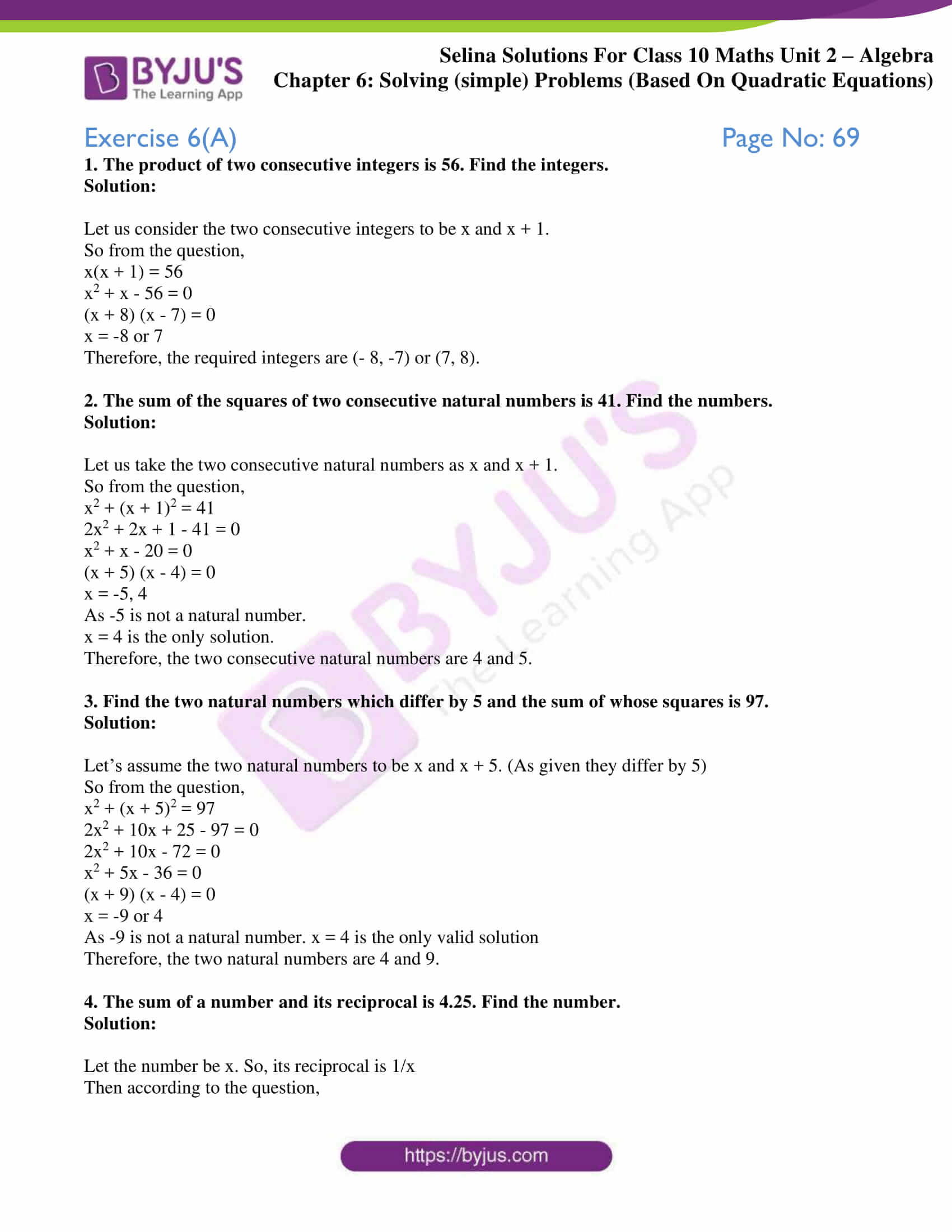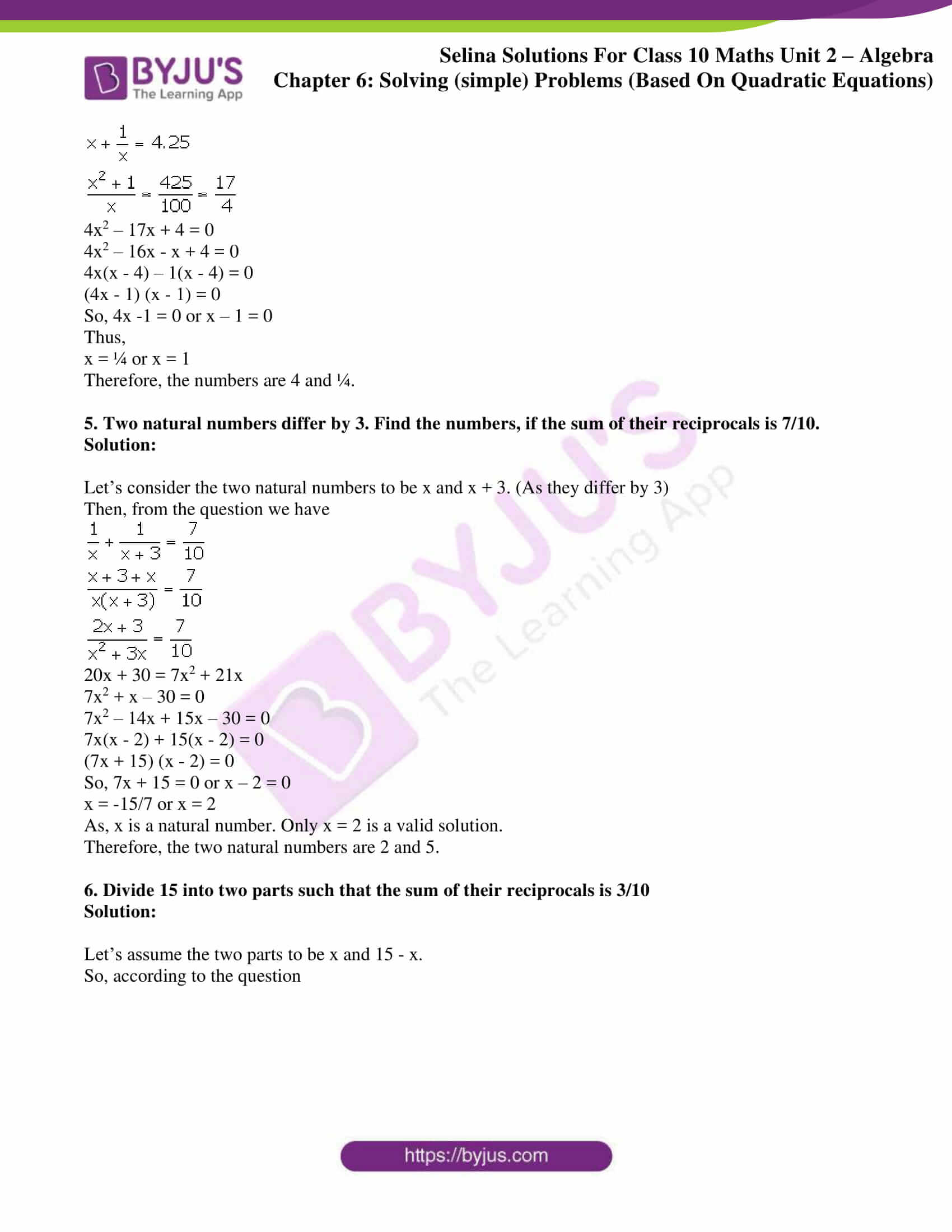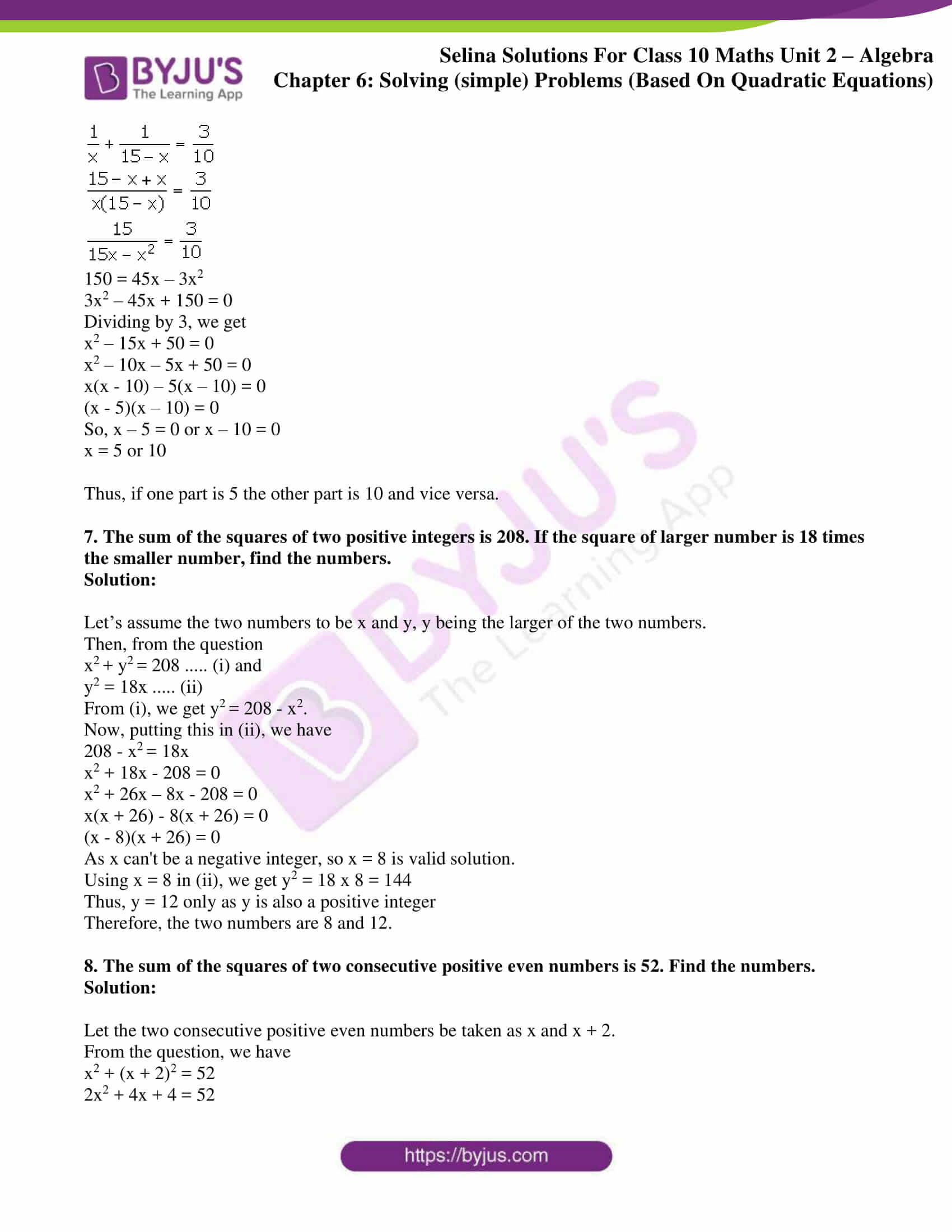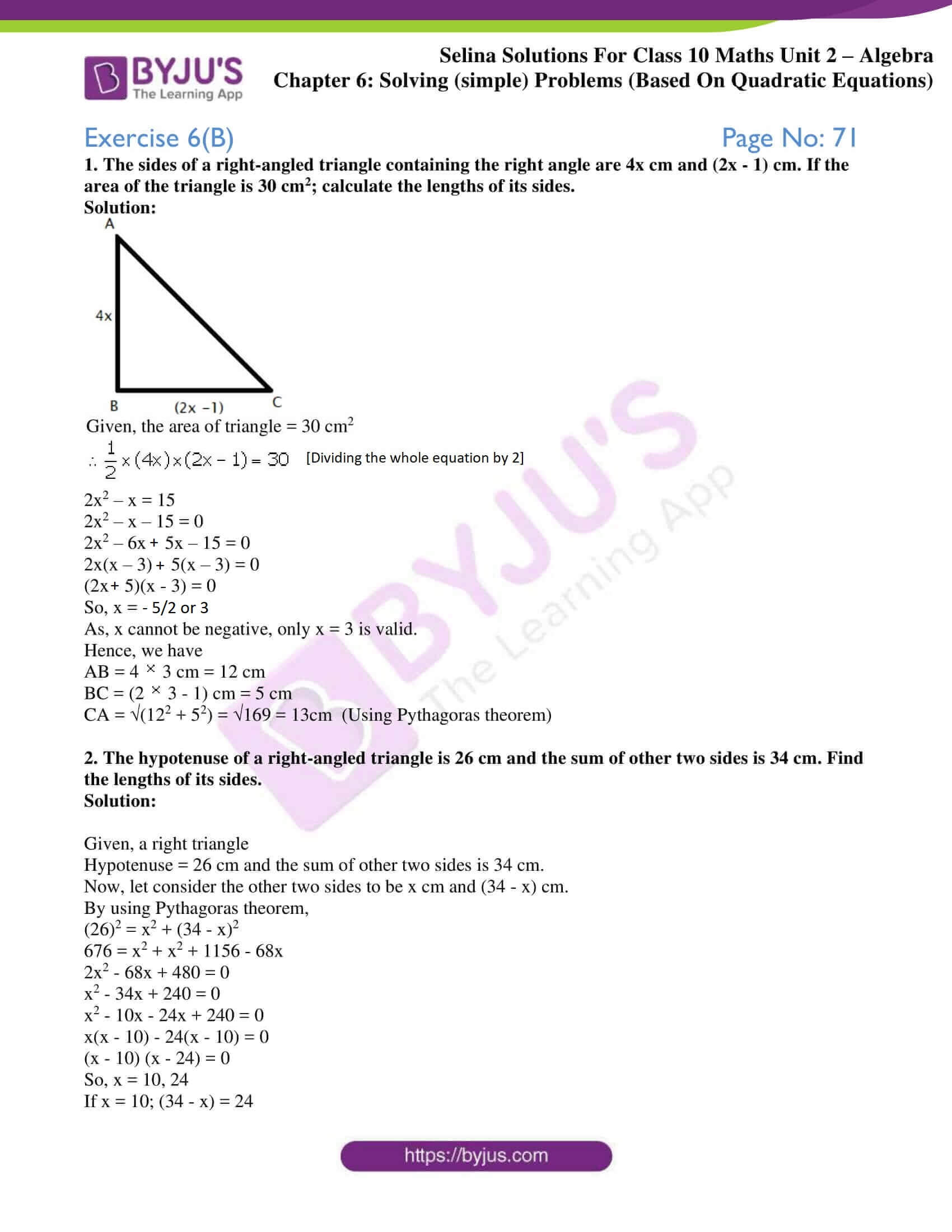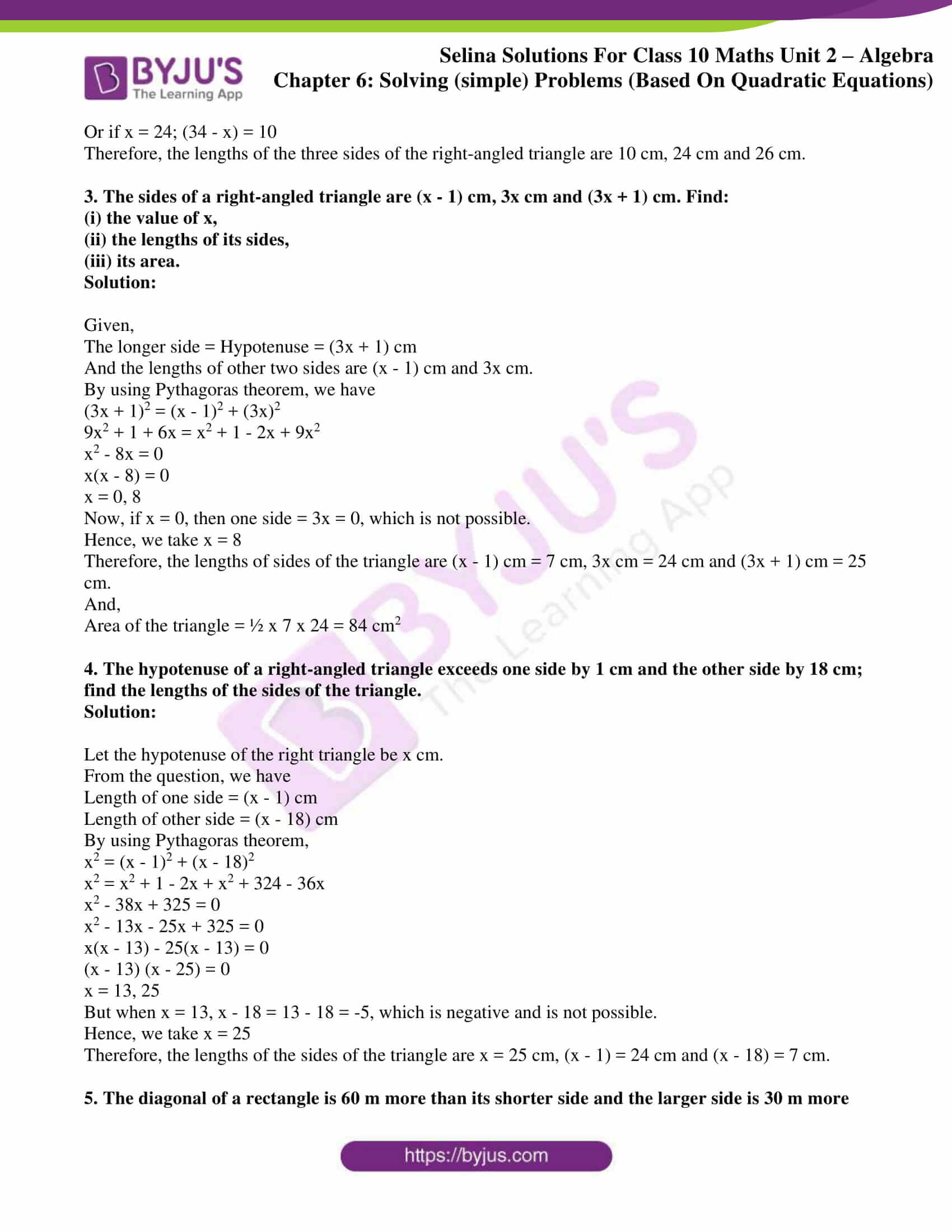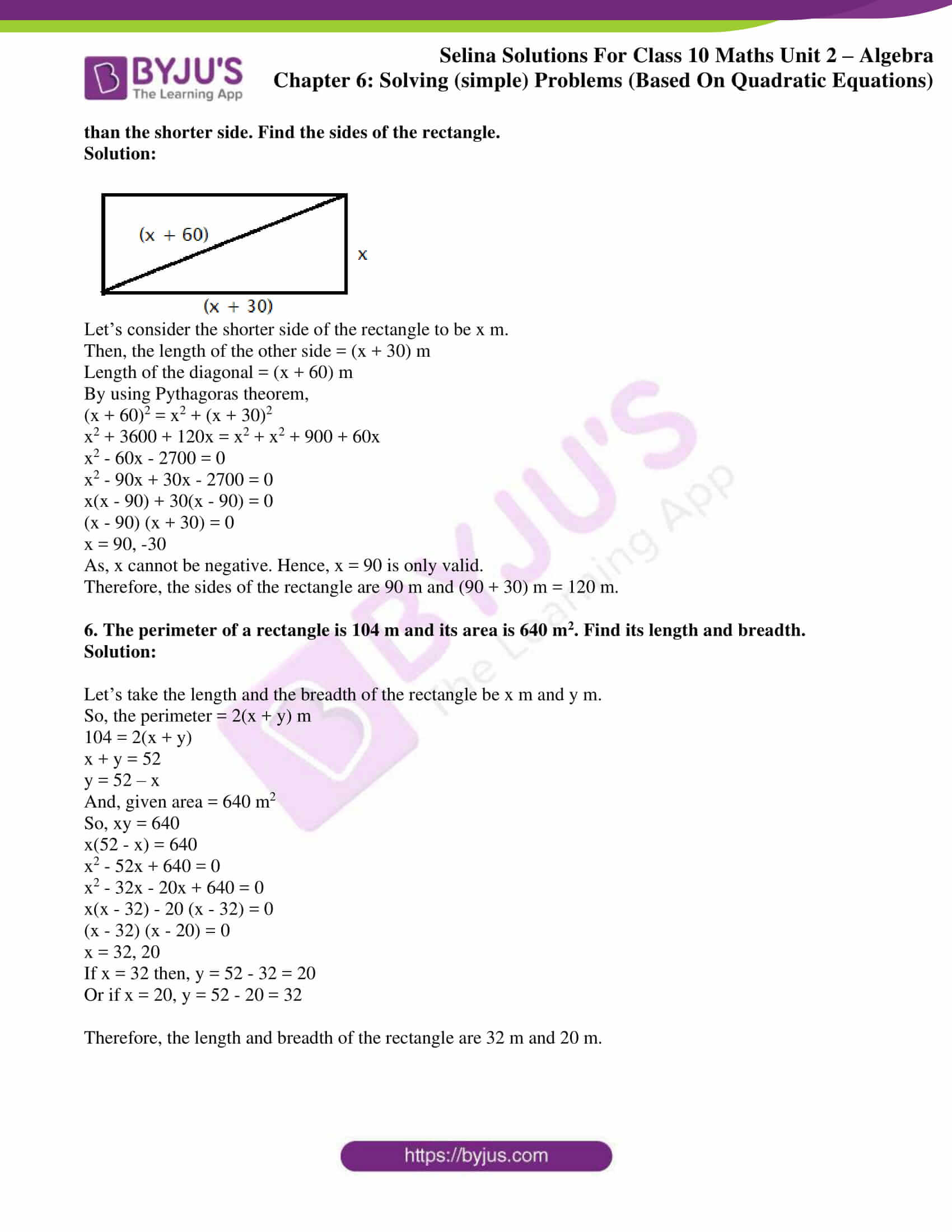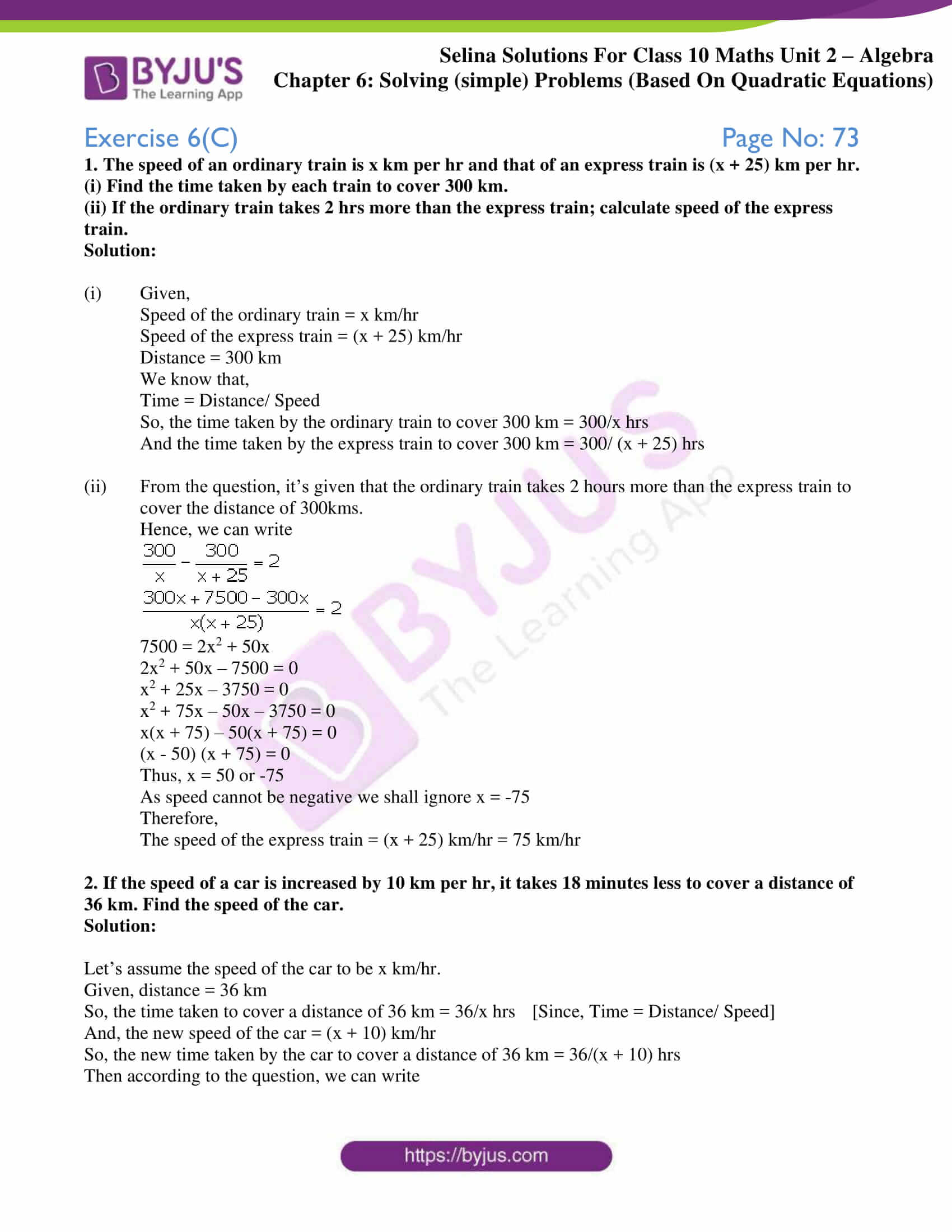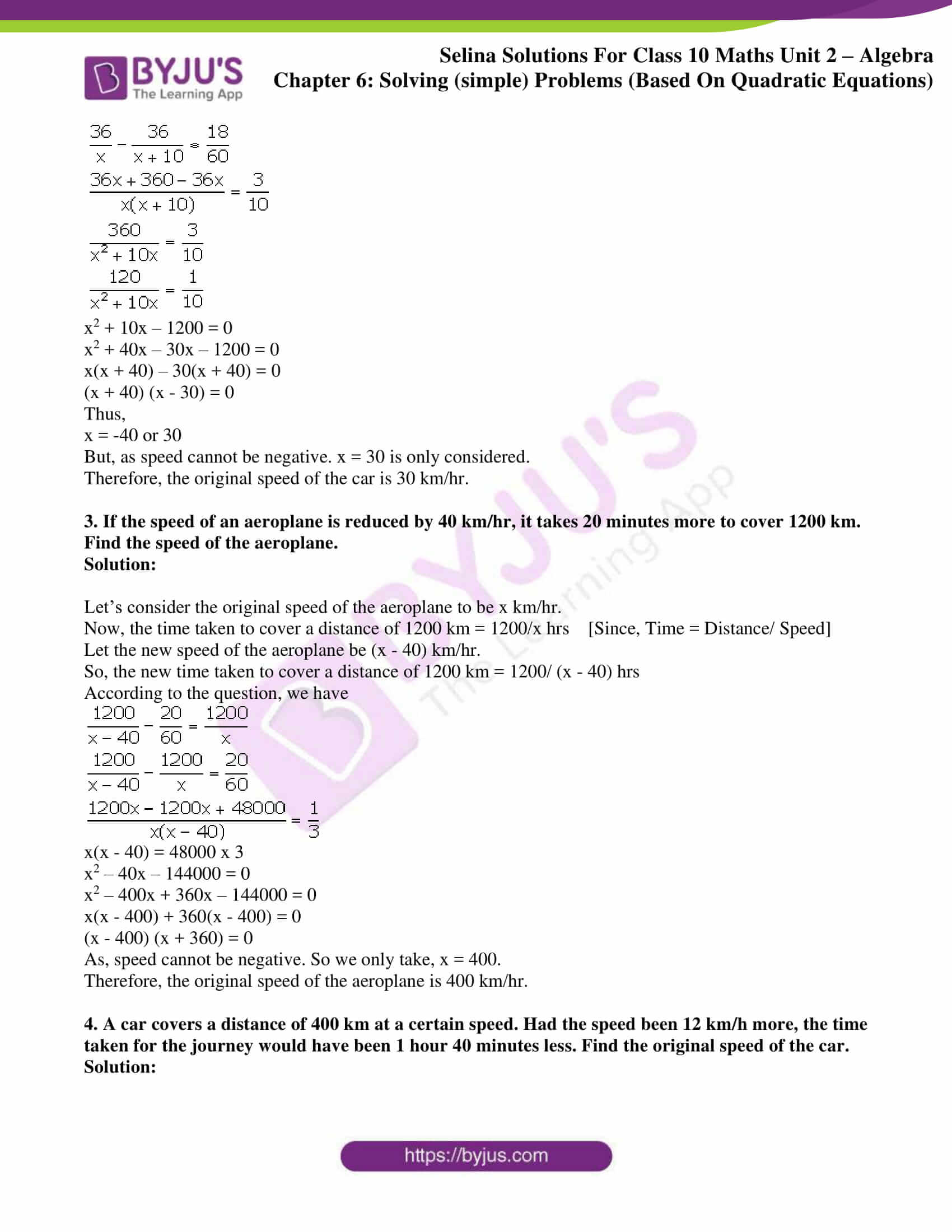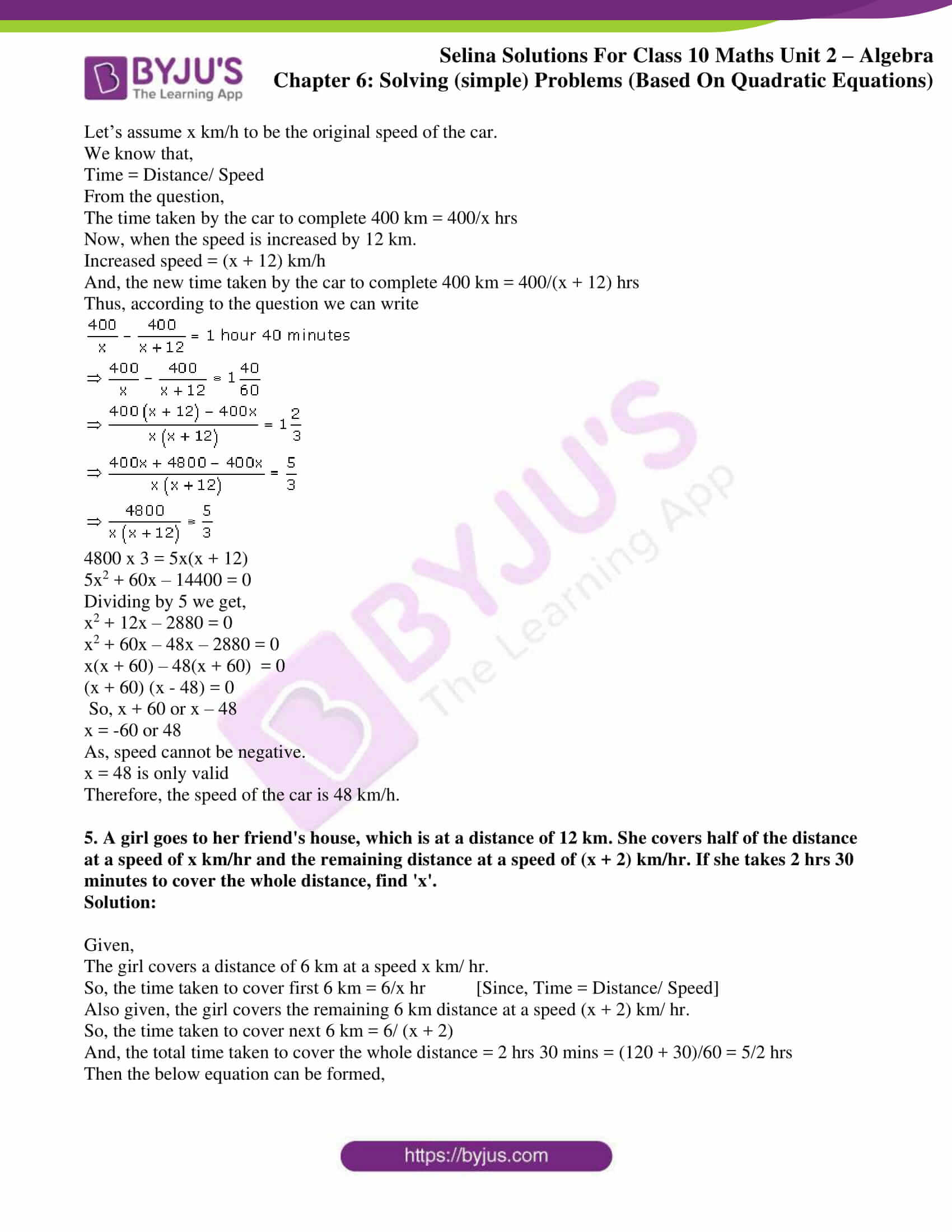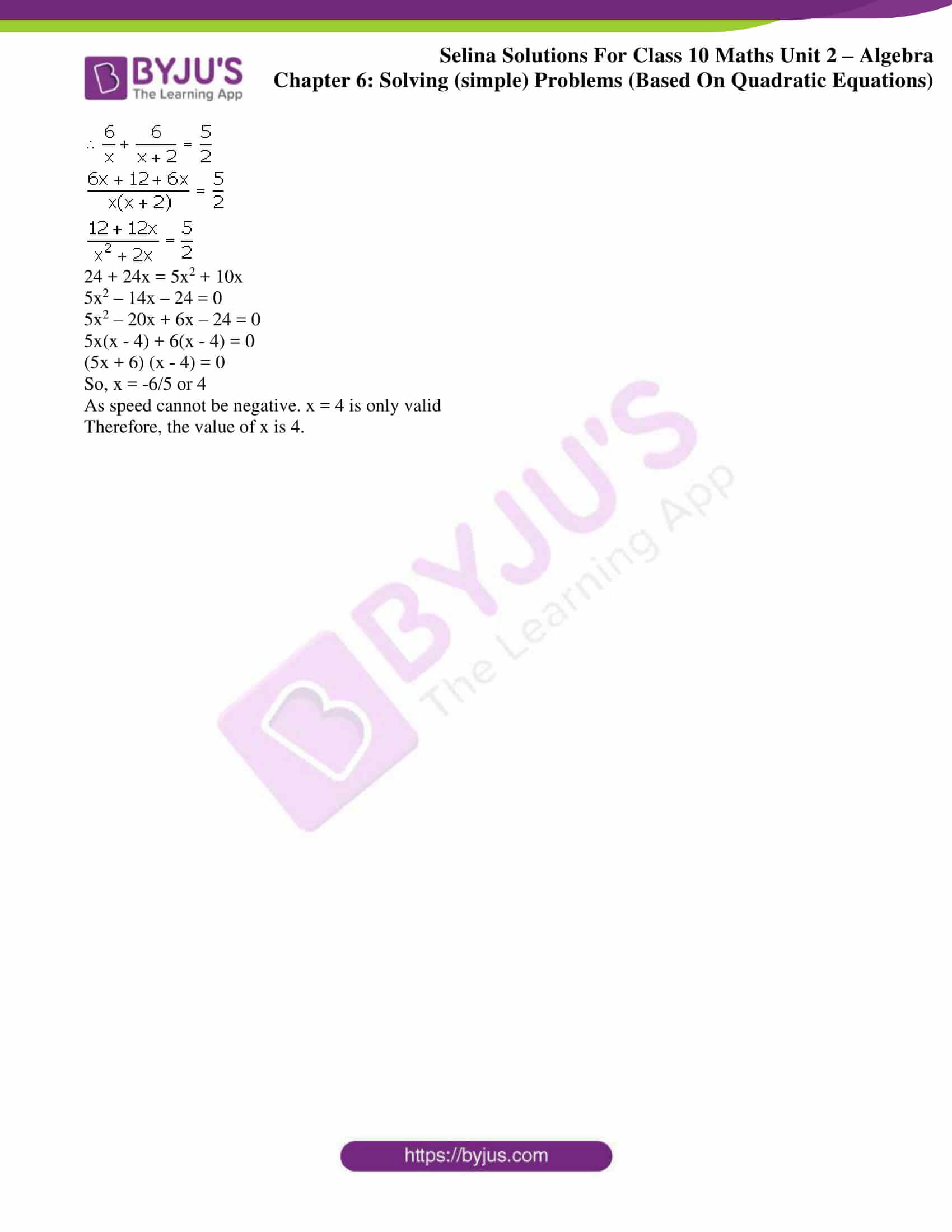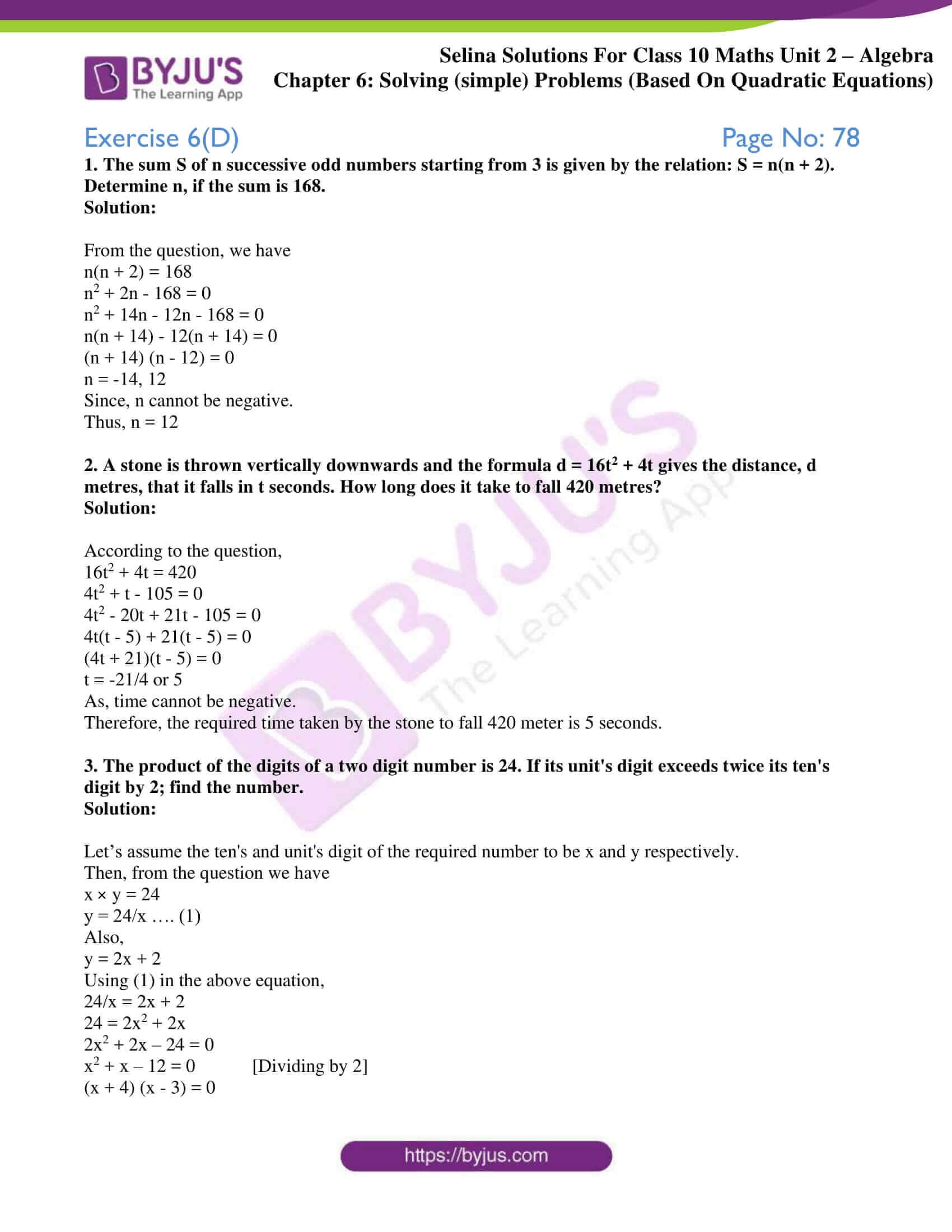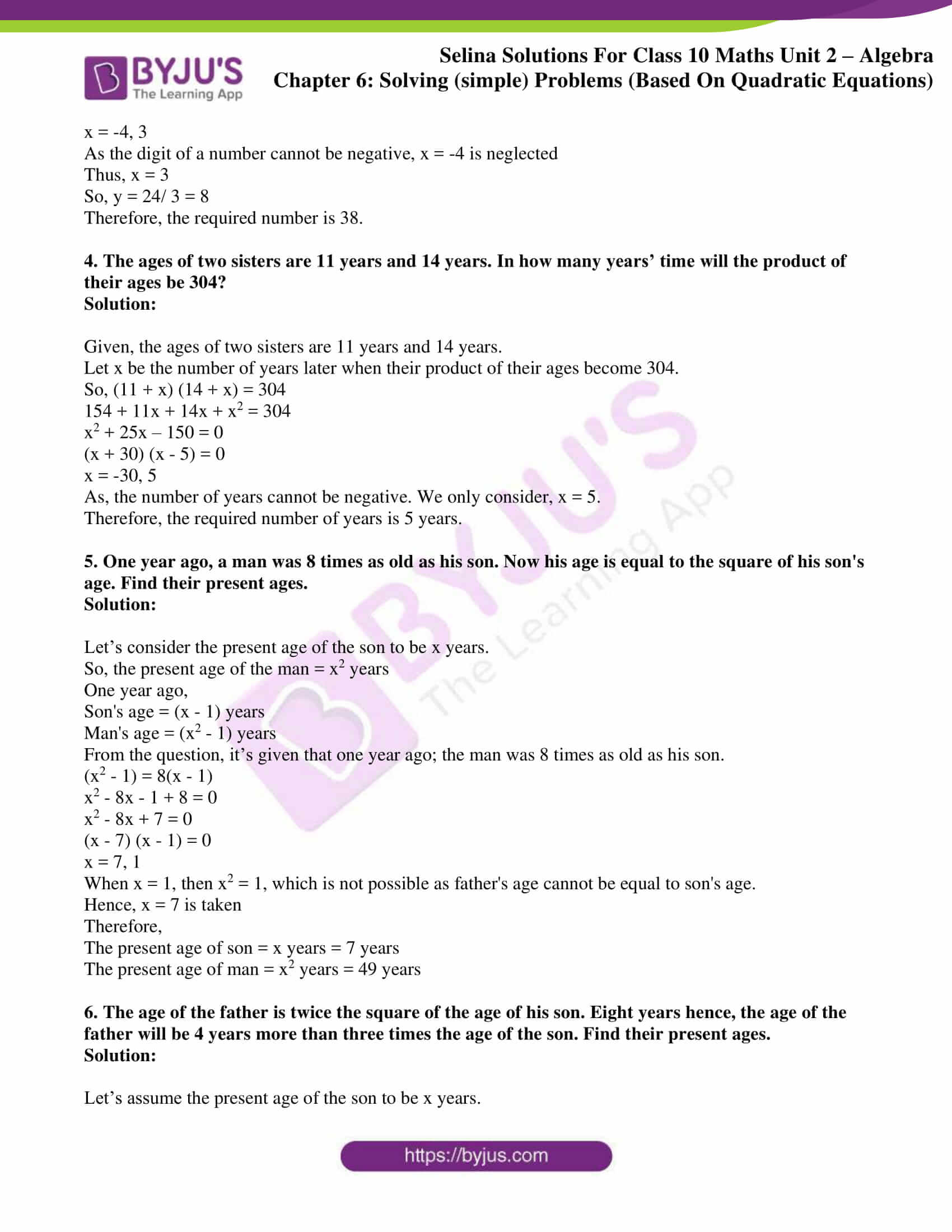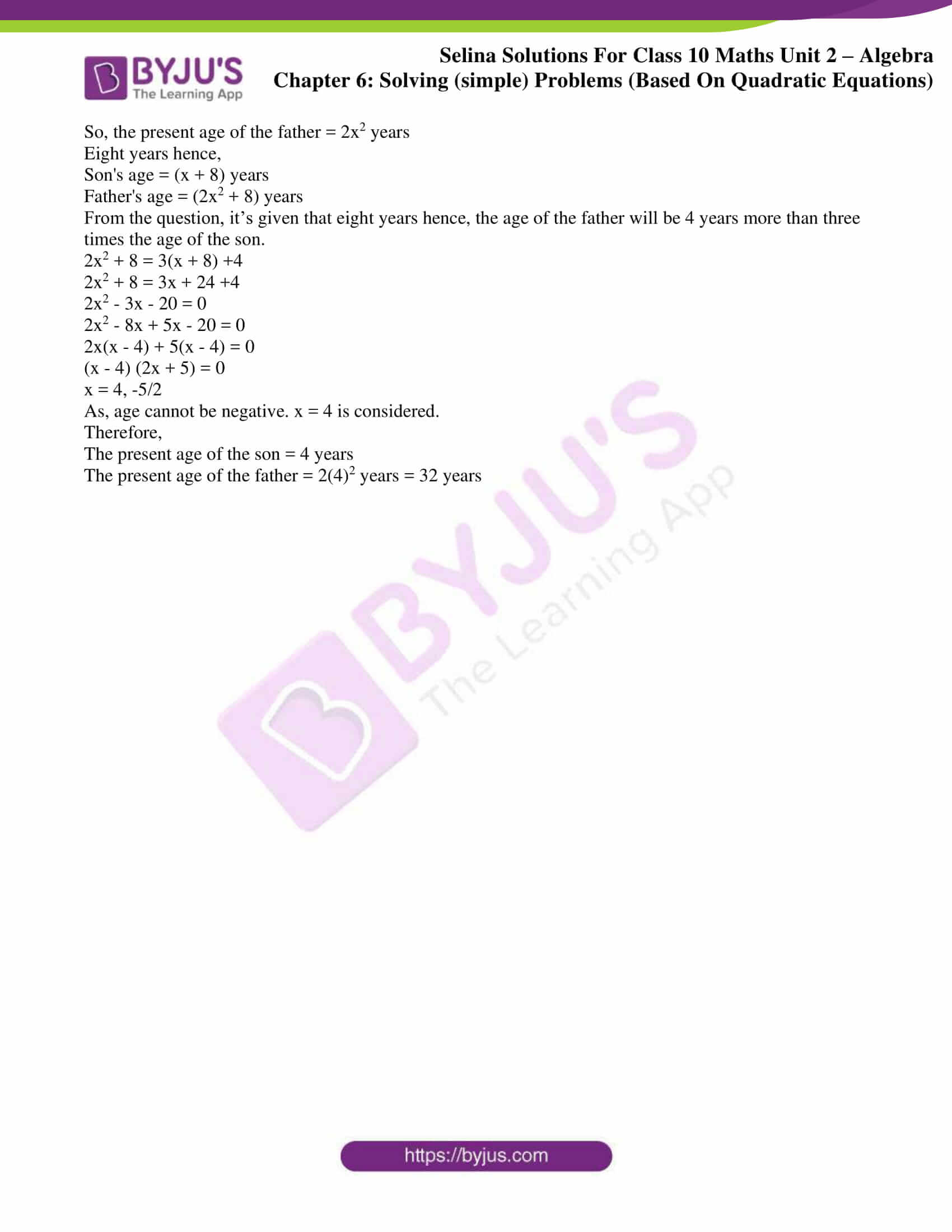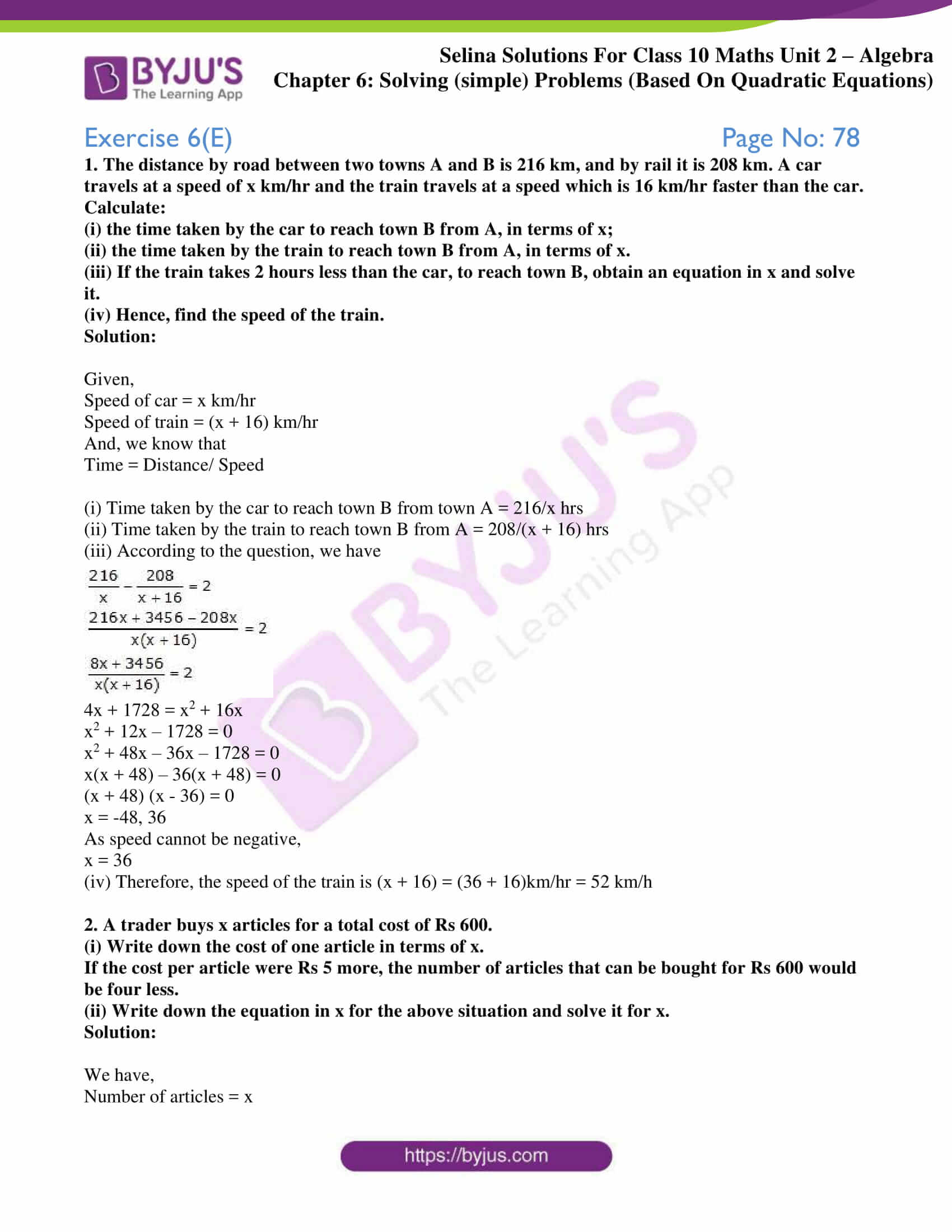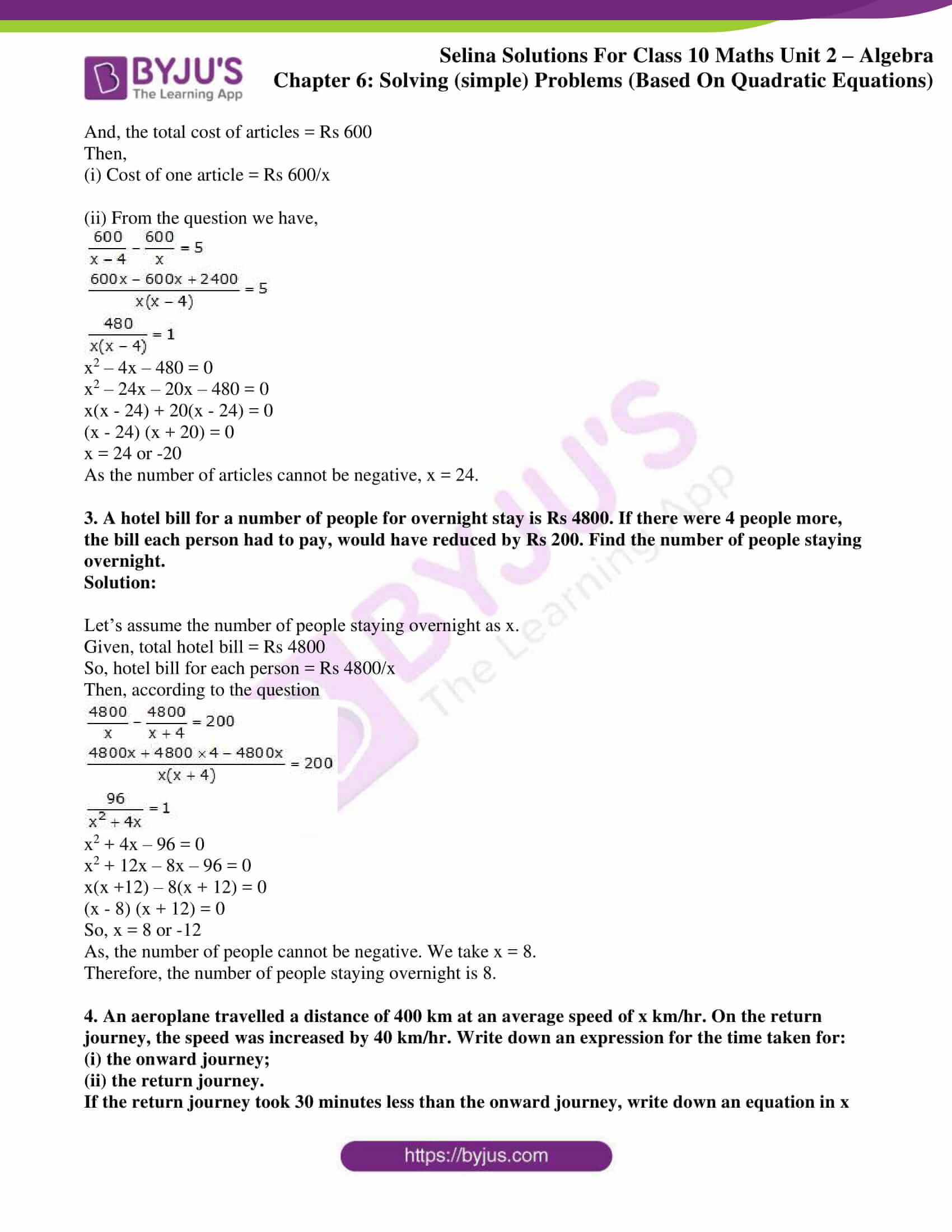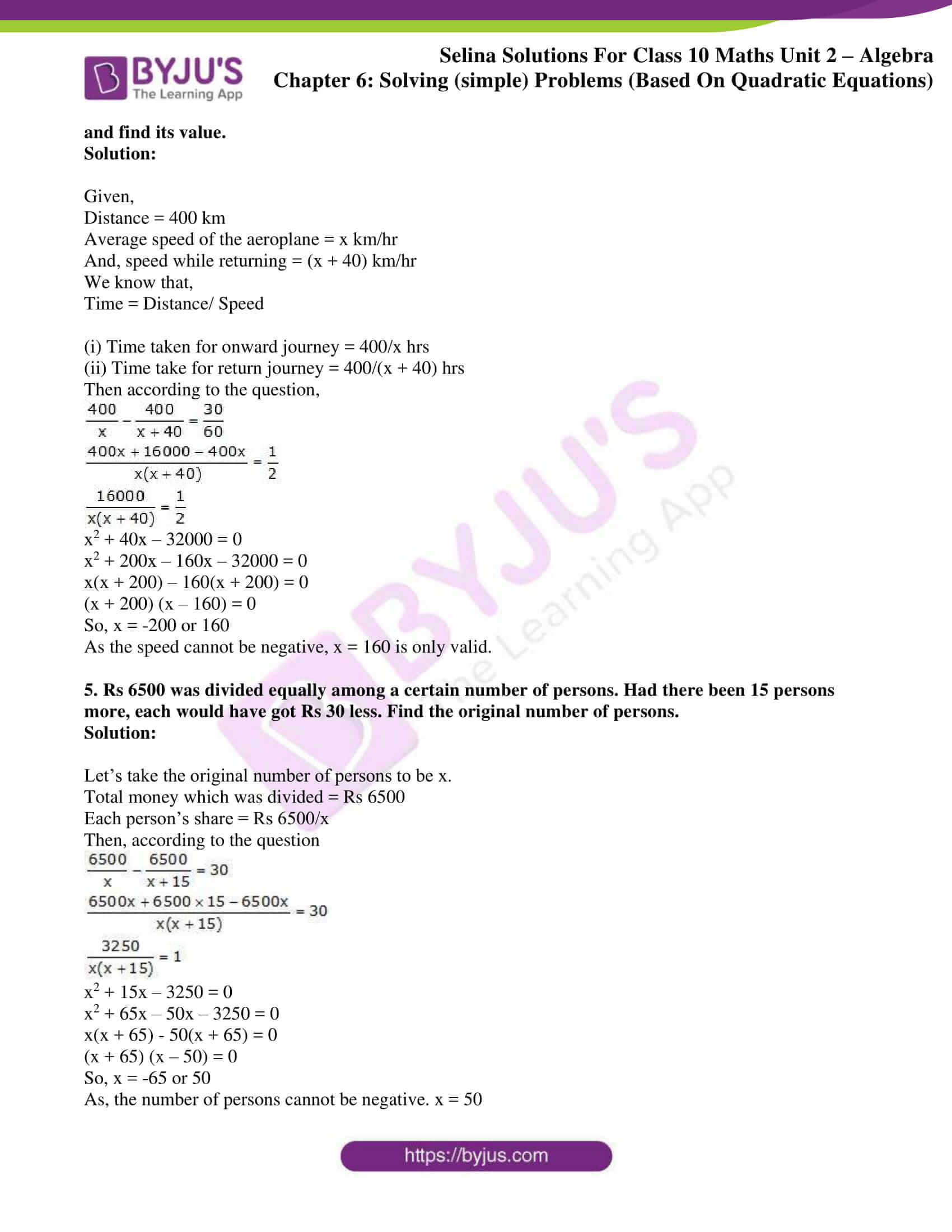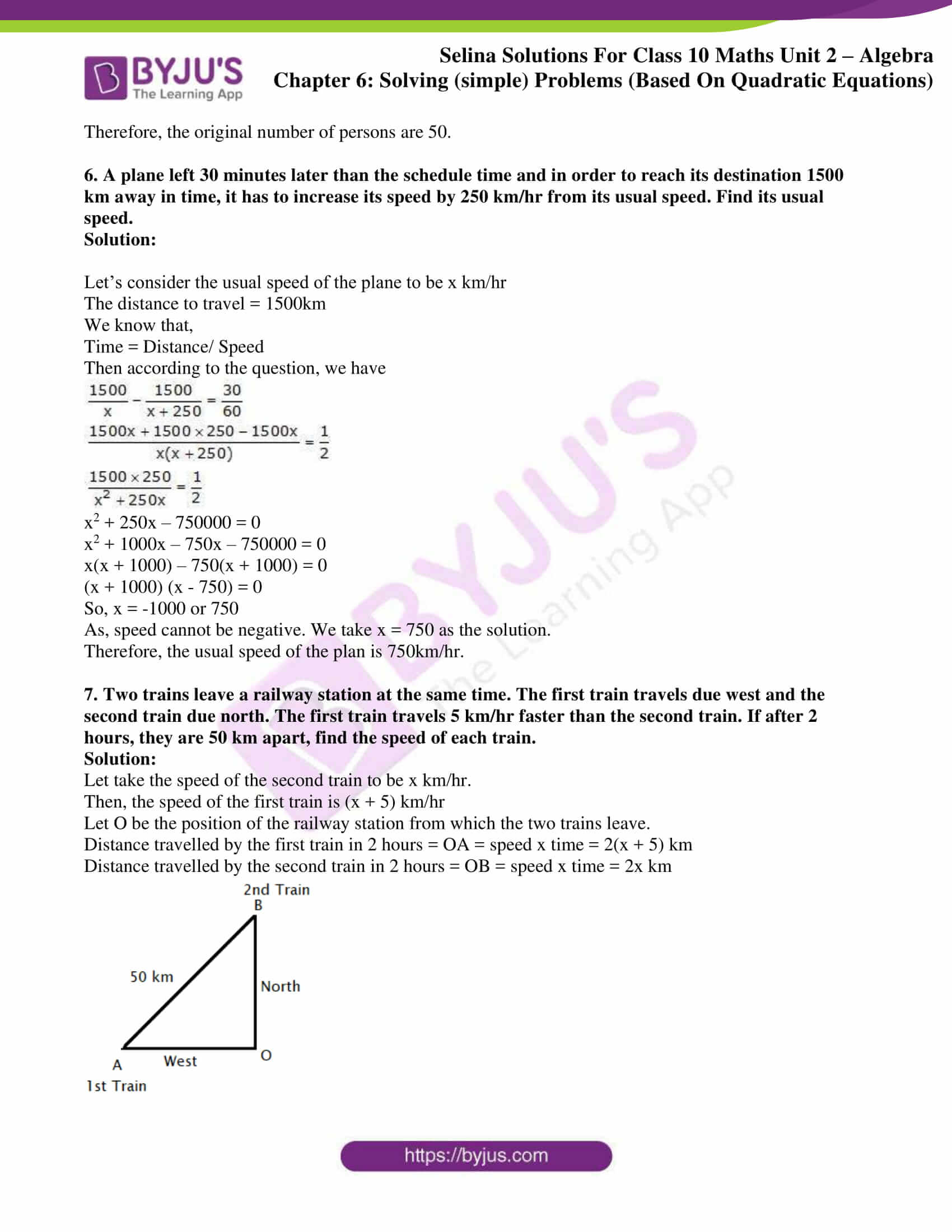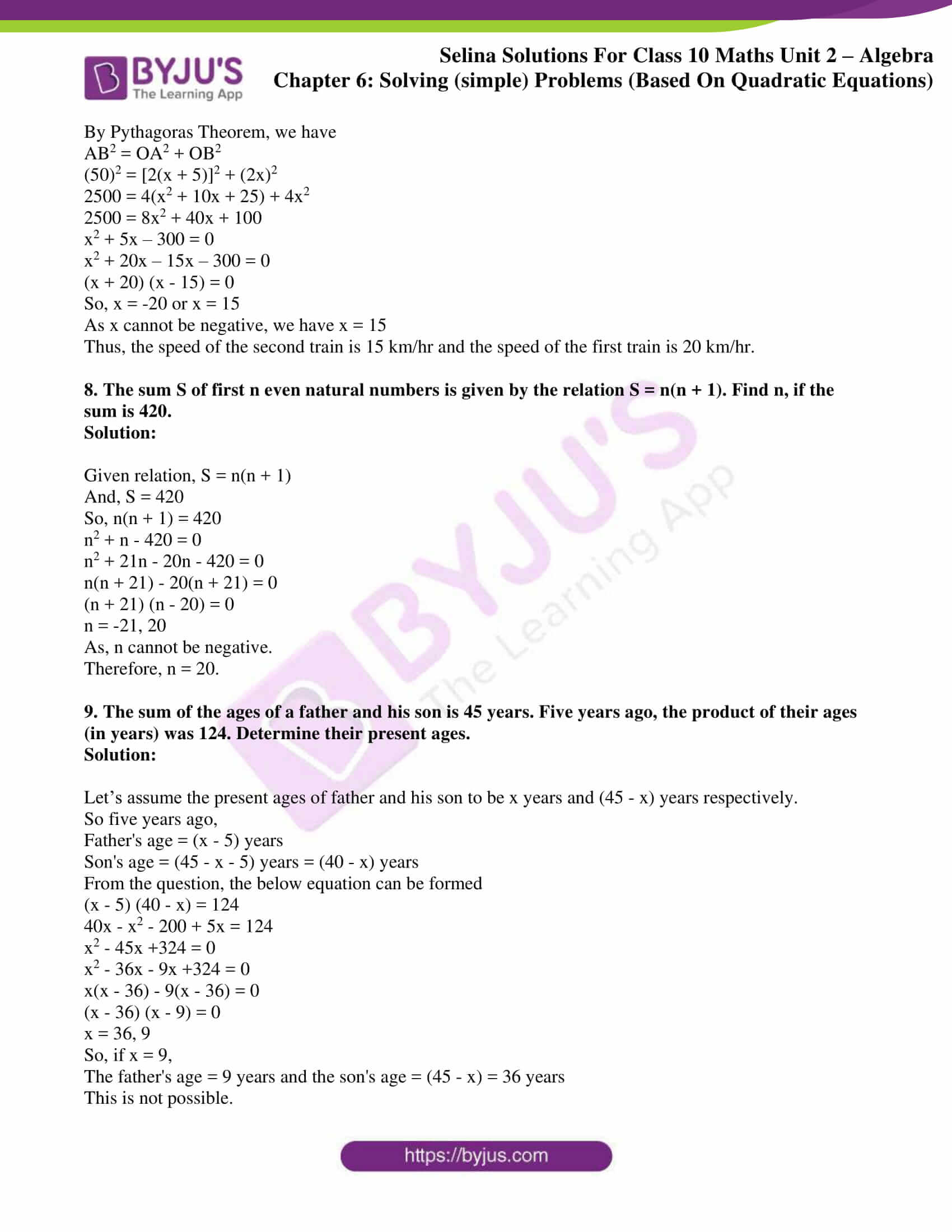### Exercises of Selina Solutions Concise Maths Class 10 Chapter 6 Solving (simple) Problems (Based On Quadratic Equations)

Exercise 6(A) Solutions

Exercise 6(B) Solutions

Exercise 6(C) Solutions

Exercise 6(D) Solutions

Exercise 6(E) Solutions

## Access Selina Solutions Concise Maths Class 10 Chapter 6 Solving (simple) Problems (Based On Quadratic Equations)

Exercise 6(A) Page No: 69

1. The product of two consecutive integers is 56. Find the integers.

Solution:

Let us consider the two consecutive integers to be x and x + 1.

So from the question,

x(x + 1) = 56

x2 + x – 56 = 0

(x + 8) (x – 7) = 0

x = -8 or 7

Therefore, the required integers are (- 8, -7) or (7, 8).

2. The sum of the squares of two consecutive natural numbers is 41. Find the numbers.

Solution:

Let us take the two consecutive natural numbers as x and x + 1.

So from the question,

x2 + (x + 1)2 = 41

2x2 + 2x + 1 – 41 = 0

x2 + x – 20 = 0

(x + 5) (x – 4) = 0

x = -5, 4

As -5 is not a natural number.

x = 4 is the only solution.

Therefore, the two consecutive natural numbers are 4 and 5.

3. Find the two natural numbers which differ by 5 and the sum of whose squares is 97.

Solution:

Let’s assume the two natural numbers to be x and x + 5. (As given they differ by 5)

So from the question,

x2 + (x + 5)2 = 97

2x2 + 10x + 25 – 97 = 0

2x2 + 10x – 72 = 0

x2 + 5x – 36 = 0

(x + 9) (x – 4) = 0

x = -9 or 4

As -9 is not a natural number. x = 4 is the only valid solution

Therefore, the two natural numbers are 4 and 9.

4. The sum of a number and its reciprocal is 4.25. Find the number.

Solution:

Let the number be x. So, its reciprocal is 1/x

Then according to the question,4x2 – 17x + 4 = 0

4x2 – 16x – x + 4 = 0

4x(x – 4) – 1(x – 4) = 0

(4x – 1) (x – 1) = 0

So, 4x -1 = 0 or x – 1 = 0

Thus,

x = ¼ or x = 1

Therefore, the numbers are 4 and ¼.

5. Two natural numbers differ by 3. Find the numbers, if the sum of their reciprocals is 7/10.

Solution:

Let’s consider the two natural numbers to be x and x + 3. (As they differ by 3)

Then, from the question we have20x + 30 = 7x2 + 21x

7x2 + x – 30 = 0

7x2 – 14x + 15x – 30 = 0

7x(x – 2) + 15(x – 2) = 0

(7x + 15) (x – 2) = 0

So, 7x + 15 = 0 or x – 2 = 0

x = -15/7 or x = 2

As, x is a natural number. Only x = 2 is a valid solution.

Therefore, the two natural numbers are 2 and 5.

6. Divide 15 into two parts such that the sum of their reciprocals is 3/10

Solution:

Let’s assume the two parts to be x and 15 – x.

So, according to the question150 = 45x – 3x2

3x2 – 45x + 150 = 0

Dividing by 3, we get

x2 – 15x + 50 = 0

x2 – 10x – 5x + 50 = 0

x(x – 10) – 5(x – 10) = 0

(x – 5)(x – 10) = 0

So, x – 5 = 0 or x – 10 = 0

x = 5 or 10

Thus, if one part is 5 the other part is 10 and vice versa.

7. The sum of the squares of two positive integers is 208. If the square of larger number is 18 times the smaller number, find the numbers.

Solution:

Let’s assume the two numbers to be x and y, y being the larger of the two numbers.

Then, from the question

x+ y= 208 ….. (i) and

y2 = 18x ….. (ii)

From (i), we get y2 = 208 – x2.

Now, putting this in (ii), we have

208 – x= 18x

x2 + 18x – 208 = 0

x2 + 26x – 8x – 208 = 0

x(x + 26) – 8(x + 26) = 0

(x – 8)(x + 26) = 0

As x can’t be a negative integer, so x = 8 is valid solution.

Using x = 8 in (ii), we get y2 = 18 x 8 = 144

Thus, y = 12 only as y is also a positive integer

Therefore, the two numbers are 8 and 12.

8. The sum of the squares of two consecutive positive even numbers is 52. Find the numbers.

Solution:

Let the two consecutive positive even numbers be taken as x and x + 2.

From the question, we have

x2 + (x + 2)2 = 52

2x2 + 4x + 4 = 52

2x2 + 4x – 48 = 0

x2 + 2x – 24 = 0

(x + 6) (x – 4) = 0

x = -6, 4

As, the numbers are positive only x = 4 is a valid solution.

Therefore, the numbers are 4 and 6.

Exercise 6(B) Page No: 71

1. The sides of a right-angled triangle containing the right angle are 4x cm and (2x – 1) cm. If the area of the triangle is 30 cm2; calculate the lengths of its sides.

Solution: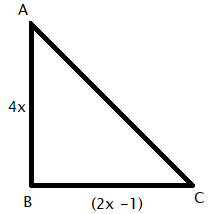Given, the area of triangle = 30 cm2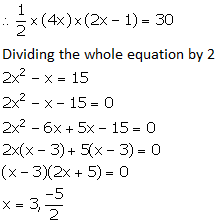As, x cannot be negative, only x = 3 is valid.

Hence, we have

AB = 4 × 3 cm = 12 cm

BC = (2 × 3 – 1) cm = 5 cm

CA = √(122 + 52) = √169 = 13cm  (Using Pythagoras theorem)

2. The hypotenuse of a right-angled triangle is 26 cm and the sum of other two sides is 34 cm. Find the lengths of its sides.

Solution:

Given, a right triangle

Hypotenuse = 26 cm and the sum of other two sides is 34 cm.

Now, let consider the other two sides to be x cm and (34 – x) cm.

By using Pythagoras theorem,

(26)2 = x2 + (34 – x)2

676 = x2 + x2 + 1156 – 68x

2x2 – 68x + 480 = 0

x2 – 34x + 240 = 0

x2 – 10x – 24x + 240 = 0

x(x – 10) – 24(x – 10) = 0

(x – 10) (x – 24) = 0

So, x = 10, 24

If x = 10; (34 – x) = 24

Or if x = 24; (34 – x) = 10

Therefore, the lengths of the three sides of the right-angled triangle are 10 cm, 24 cm and 26 cm.

3. The sides of a right-angled triangle are (x – 1) cm, 3x cm and (3x + 1) cm. Find:

(i) the value of x,

(ii) the lengths of its sides,

(iii) its area.

Solution:

Given,

The longer side = Hypotenuse = (3x + 1) cm

And the lengths of other two sides are (x – 1) cm and 3x cm.

By using Pythagoras theorem, we have

(3x + 1)2 = (x – 1)2 + (3x)2

9x2 + 1 + 6x = x2 + 1 – 2x + 9x2

x2 – 8x = 0

x(x – 8) = 0

x = 0, 8

Now, if x = 0, then one side = 3x = 0, which is not possible.

Hence, we take x = 8

Therefore, the lengths of sides of the triangle are (x – 1) cm = 7 cm, 3x cm = 24 cm and (3x + 1) cm = 25 cm.

And,

Area of the triangle = ½ x 7 x 24 = 84 cm2

4. The hypotenuse of a right-angled triangle exceeds one side by 1 cm and the other side by 18 cm; find the lengths of the sides of the triangle.

Solution:

Let the hypotenuse of the right triangle be x cm.

From the question, we have

Length of one side = (x – 1) cm

Length of other side = (x – 18) cm

By using Pythagoras theorem,

x2 = (x – 1)2 + (x – 18)2

x2 = x2 + 1 – 2x + x2 + 324 – 36x

x2 – 38x + 325 = 0

x2 – 13x – 25x + 325 = 0

x(x – 13) – 25(x – 13) = 0

(x – 13) (x – 25) = 0

x = 13, 25

But when x = 13, x – 18 = 13 – 18 = -5, which is negative and is not possible.

Hence, we take x = 25

Therefore, the lengths of the sides of the triangle are x = 25 cm, (x – 1) = 24 cm and (x – 18) = 7 cm.

5. The diagonal of a rectangle is 60 m more than its shorter side and the larger side is 30 m more than the shorter side. Find the sides of the rectangle.

Solution: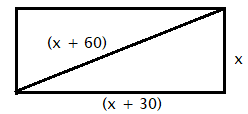Let’s consider the shorter side of the rectangle to be x m.

Then, the length of the other side = (x + 30) m

Length of the diagonal = (x + 60) m

By using Pythagoras theorem,

(x + 60)2 = x2 + (x + 30)2

x2 + 3600 + 120x = x2 + x2 + 900 + 60x

x2 – 60x – 2700 = 0

x2 – 90x + 30x – 2700 = 0

x(x – 90) + 30(x – 90) = 0

(x – 90) (x + 30) = 0

x = 90, -30

As, x cannot be negative. Hence, x = 90 is only valid.

Therefore, the sides of the rectangle are 90 m and (90 + 30) m = 120 m.

6. The perimeter of a rectangle is 104 m and its area is 640 m2. Find its length and breadth.

Solution:

Let’s take the length and the breadth of the rectangle be x m and y m.

So, the perimeter = 2(x + y) m

104 = 2(x + y)

x + y = 52

y = 52 – x

And, given area = 640 m2

So, xy = 640

x(52 – x) = 640

x2 – 52x + 640 = 0

x2 – 32x – 20x + 640 = 0

x(x – 32) – 20 (x – 32) = 0

(x – 32) (x – 20) = 0

x = 32, 20

If x = 32 then, y = 52 – 32 = 20

Or if x = 20, y = 52 – 20 = 32

Therefore, the length and breadth of the rectangle are 32 m and 20 m.

Exercise 6(C) Page No: 73

1. The speed of an ordinary train is x km per hr and that of an express train is (x + 25) km per hr.

(i) Find the time taken by each train to cover 300 km.

(ii) If the ordinary train takes 2 hrs more than the express train; calculate speed of the express train.

Solution:

(i) Given,

Speed of the ordinary train = x km/hr

Speed of the express train = (x + 25) km/hr

Distance = 300 km

We know that,

Time = Distance/ Speed

So, the time taken by the ordinary train to cover 300 km = 300/x hrs

And the time taken by the express train to cover 300 km = 300/ (x + 25) hrs

(ii) From the question, it’s given that the ordinary train takes 2 hours more than the express train to cover the distance of 300kms.

Hence, we can write7500 = 2x2 + 50x

2x2 + 50x – 7500 = 0

x2 + 25x – 3750 = 0

x2 + 75x – 50x – 3750 = 0

x(x + 75) – 50(x + 75) = 0

(x – 50) (x + 75) = 0

Thus, x = 50 or -75

As speed cannot be negative we shall ignore x = -75

Therefore,

The speed of the express train = (x + 25) km/hr = 75 km/hr

2. If the speed of a car is increased by 10 km per hr, it takes 18 minutes less to cover a distance of 36 km. Find the speed of the car.

Solution:

Let’s assume the speed of the car to be x km/hr.

Given, distance = 36 km

So, the time taken to cover a distance of 36 km = 36/x hrs  [Since, Time = Distance/ Speed]

And, the new speed of the car = (x + 10) km/hr

So, the new time taken by the car to cover a distance of 36 km = 36/(x + 10) hrs

Then according to the question, we can writex2 + 10x – 1200 = 0

x2 + 40x – 30x – 1200 = 0

x(x + 40) – 30(x + 40) = 0

(x + 40) (x – 30) = 0

Thus,

x = -40 or 30

But, as speed cannot be negative. x = 30 is only considered.

Therefore, the original speed of the car is 30 km/hr.

3. If the speed of an aeroplane is reduced by 40 km/hr, it takes 20 minutes more to cover 1200 km. Find the speed of the aeroplane.

Solution:

Let’s consider the original speed of the aeroplane to be x km/hr.

Now, the time taken to cover a distance of 1200 km = 1200/x hrs  [Since, Time = Distance/ Speed]

Let the new speed of the aeroplane be (x – 40) km/hr.

So, the new time taken to cover a distance of 1200 km = 1200/ (x – 40) hrs

According to the question, we havex(x – 40) = 48000 x 3

x2 – 40x – 144000 = 0

x2 – 400x + 360x – 144000 = 0

x(x – 400) + 360(x – 400) = 0

(x – 400) (x + 360) = 0

As, speed cannot be negative. So we only take, x = 400.

Therefore, the original speed of the aeroplane is 400 km/hr.

4. A car covers a distance of 400 km at a certain speed. Had the speed been 12 km/h more, the time taken for the journey would have been 1 hour 40 minutes less. Find the original speed of the car.

Solution:

Let’s assume x km/h to be the original speed of the car.

We know that,

Time = Distance/ Speed

From the question,

The time taken by the car to complete 400 km = 400/x hrs

Now, when the speed is increased by 12 km.

Increased speed = (x + 12) km/h

And, the new time taken by the car to complete 400 km = 400/(x + 12) hrs

Thus, according to the question we can write4800 x 3 = 5x(x + 12)

5x2 + 60x – 14400 = 0

Dividing by 5 we get,

x2 + 12x – 2880 = 0

x2 + 60x – 48x – 2880 = 0

x(x + 60) – 48(x + 60) = 0

(x + 60) (x – 48) = 0

So, x + 60 or x – 48

x = -60 or 48

As, speed cannot be negative.

x = 48 is only valid

Therefore, the speed of the car is 48 km/h.

5. A girl goes to her friend’s house, which is at a distance of 12 km. She covers half of the distance at a speed of x km/hr and the remaining distance at a speed of (x + 2) km/hr. If she takes 2 hrs 30 minutes to cover the whole distance, find ‘x’.

Solution:

Given,

The girl covers a distance of 6 km at a speed x km/ hr.

So, the time taken to cover first 6 km = 6/x hr [Since, Time = Distance/ Speed]

Also given, the girl covers the remaining 6 km distance at a speed (x + 2) km/ hr.

So, the time taken to cover next 6 km = 6/ (x + 2)

And, the total time taken to cover the whole distance = 2 hrs 30 mins = (120 + 30)/60 = 5/2 hrs

Then the below equation can be formed,24 + 24x = 5x2 + 10x

5x2 – 14x – 24 = 0

5x2 – 20x + 6x – 24 = 0

5x(x – 4) + 6(x – 4) = 0

(5x + 6) (x – 4) = 0

So, x = -6/5 or 4

As speed cannot be negative. x = 4 is only valid

Therefore, the value of x is 4.

Exercise 6(D) Page No: 78

1. The sum S of n successive odd numbers starting from 3 is given by the relation: S = n(n + 2). Determine n, if the sum is 168.

Solution:

From the question, we have

n(n + 2) = 168

n2 + 2n – 168 = 0

n2 + 14n – 12n – 168 = 0

n(n + 14) – 12(n + 14) = 0

(n + 14) (n – 12) = 0

n = -14, 12

Since, n cannot be negative.

Thus, n = 12

2. A stone is thrown vertically downwards and the formula d = 16t2 + 4t gives the distance, d metres, that it falls in t seconds. How long does it take to fall 420 metres?

Solution:

According to the question,

16t2 + 4t = 420

4t2 + t – 105 = 0

4t2 – 20t + 21t – 105 = 0

4t(t – 5) + 21(t – 5) = 0

(4t + 21)(t – 5) = 0

t = -21/4 or 5

As, time cannot be negative.

Therefore, the required time taken by the stone to fall 420 meter is 5 seconds.

3. The product of the digits of a two digit number is 24. If its unit’s digit exceeds twice its ten’s digit by 2; find the number.

Solution:

Let’s assume the ten’s and unit’s digit of the required number to be x and y respectively.

Then, from the question we have

x × y = 24

y = 24/x …. (1)

Also,

y = 2x + 2

Using (1) in the above equation,

24/x = 2x + 2

24 = 2x2 + 2x

2x2 + 2x – 24 = 0

x2 + x – 12 = 0 [Dividing by 2]

(x + 4) (x – 3) = 0

x = -4, 3

As the digit of a number cannot be negative, x = -4 is neglected

Thus, x = 3

So, y = 24/ 3 = 8

Therefore, the required number is 38.

4. The ages of two sisters are 11 years and 14 years. In how many years’ time will the product of their ages be 304?

Solution:

Given, the ages of two sisters are 11 years and 14 years.

Let x be the number of years later when their product of their ages become 304.

So, (11 + x) (14 + x) = 304

154 + 11x + 14x + x2 = 304

x2 + 25x – 150 = 0

(x + 30) (x – 5) = 0

x = -30, 5

As, the number of years cannot be negative. We only consider, x = 5.

Therefore, the required number of years is 5 years.

5. One year ago, a man was 8 times as old as his son. Now his age is equal to the square of his son’s age. Find their present ages.

Solution:

Let’s consider the present age of the son to be x years.

So, the present age of the man = x2 years

One year ago,

Son’s age = (x – 1) years

Man’s age = (x2 – 1) years

From the question, it’s given that one year ago; the man was 8 times as old as his son.

(x2 – 1) = 8(x – 1)

x2 – 8x – 1 + 8 = 0

x2 – 8x + 7 = 0

(x – 7) (x – 1) = 0

x = 7, 1

When x = 1, then x2 = 1, which is not possible as father’s age cannot be equal to son’s age.

Hence, x = 7 is taken

Therefore,

The present age of son = x years = 7 years

The present age of man = x2 years = 49 years

6. The age of the father is twice the square of the age of his son. Eight years hence, the age of the father will be 4 years more than three times the age of the son. Find their present ages.

Solution:

Let’s assume the present age of the son to be x years.

So, the present age of the father = 2x2 years

Eight years hence,

Son’s age = (x + 8) years

Father’s age = (2x2 + 8) years

From the question, it’s given that eight years hence, the age of the father will be 4 years more than three times the age of the son.

2x2 + 8 = 3(x + 8) +4

2x2 + 8 = 3x + 24 +4

2x2 – 3x – 20 = 0

2x2 – 8x + 5x – 20 = 0

2x(x – 4) + 5(x – 4) = 0

(x – 4) (2x + 5) = 0

x = 4, -5/2

As, age cannot be negative. x = 4 is considered.

Therefore,

The present age of the son = 4 years

The present age of the father = 2(4)2 years = 32 years

Exercise 6(E) Page No: 78

1. The distance by road between two towns A and B is 216 km, and by rail it is 208 km. A car travels at a speed of x km/hr and the train travels at a speed which is 16 km/hr faster than the car. Calculate:

(i) the time taken by the car to reach town B from A, in terms of x;

(ii) the time taken by the train to reach town B from A, in terms of x.

(iii) If the train takes 2 hours less than the car, to reach town B, obtain an equation in x and solve it.

(iv) Hence, find the speed of the train.

Solution:

Given,

Speed of car = x km/hr

Speed of train = (x + 16) km/hr

And, we know that

Time = Distance/ Speed

(i) Time taken by the car to reach town B from town A = 216/x hrs

(ii) Time taken by the train to reach town B from A = 208/(x + 16) hrs

(iii) According to the question, we have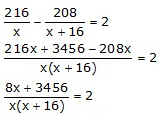4x + 1728 = x2 + 16x

x2 + 12x – 1728 = 0

x2 + 48x – 36x – 1728 = 0

x(x + 48) – 36(x + 48) = 0

(x + 48) (x – 36) = 0

x = -48, 36

As speed cannot be negative,

x = 36

(iv) Therefore, the speed of the train is (x + 16) = (36 + 16)km/hr = 52 km/h

2. A trader buys x articles for a total cost of Rs 600.

(i) Write down the cost of one article in terms of x.

If the cost per article were Rs 5 more, the number of articles that can be bought for Rs 600 would be four less.

(ii) Write down the equation in x for the above situation and solve it for x.

Solution:

We have,

Number of articles = x

And, the total cost of articles = Rs 600

Then,

(i) Cost of one article = Rs 600/x

(ii) From the question we have,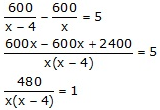x2 – 4x – 480 = 0

x2 – 24x – 20x – 480 = 0

x(x – 24) + 20(x – 24) = 0

(x – 24) (x + 20) = 0

x = 24 or -20

As the number of articles cannot be negative, x = 24.

3. A hotel bill for a number of people for overnight stay is Rs 4800. If there were 4 people more, the bill each person had to pay, would have reduced by Rs 200. Find the number of people staying overnight.

Solution:

Let’s assume the number of people staying overnight as x.

Given, total hotel bill = Rs 4800

So, hotel bill for each person = Rs 4800/x

Then, according to the question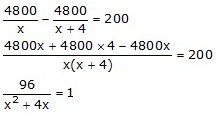x2 + 4x – 96 = 0

x2 + 12x – 8x – 96 = 0

x(x +12) – 8(x + 12) = 0

(x – 8) (x + 12) = 0

So, x = 8 or -12

As, the number of people cannot be negative. We take x = 8.

Therefore, the number of people staying overnight is 8.

4. An aeroplane travelled a distance of 400 km at an average speed of x km/hr. On the return journey, the speed was increased by 40 km/hr. Write down an expression for the time taken for:

(i) the onward journey;

(ii) the return journey.

If the return journey took 30 minutes less than the onward journey, write down an equation in x and find its value.

Solution:

Given,

Distance = 400 km

Average speed of the aeroplane = x km/hr

And, speed while returning = (x + 40) km/hr

We know that,

Time = Distance/ Speed

(i) Time taken for onward journey = 400/x hrs

(ii) Time take for return journey = 400/(x + 40) hrs

Then according to the question,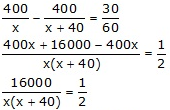x2 + 40x – 32000 = 0

x2 + 200x – 160x – 32000 = 0

x(x + 200) – 160(x + 200) = 0

(x + 200) (x – 160) = 0

So, x = -200 or 160

As the speed cannot be negative, x = 160 is only valid.

5. Rs 6500 was divided equally among a certain number of persons. Had there been 15 persons more, each would have got Rs 30 less. Find the original number of persons.

Solution:

Let’s take the original number of persons to be x.

Total money which was divided = Rs 6500

Each person’s share = Rs 6500/x

Then, according to the question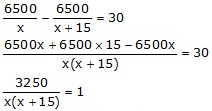x2 + 15x – 3250 = 0

x2 + 65x – 50x – 3250 = 0

x(x + 65) – 50(x + 65) = 0

(x + 65) (x – 50) = 0

So, x = -65 or 50

As, the number of persons cannot be negative. x = 50

Therefore, the original number of persons are 50.

6. A plane left 30 minutes later than the schedule time and in order to reach its destination 1500 km away in time, it has to increase its speed by 250 km/hr from its usual speed. Find its usual speed.

Solution:

Let’s consider the usual speed of the plane to be x km/hr

The distance to travel = 1500km

We know that,

Time = Distance/ Speed

Then according to the question, we have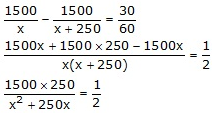x2 + 250x – 750000 = 0

x2 + 1000x – 750x – 750000 = 0

x(x + 1000) – 750(x + 1000) = 0

(x + 1000) (x – 750) = 0

So, x = -1000 or 750

As, speed cannot be negative. We take x = 750 as the solution.

Therefore, the usual speed of the plan is 750km/hr.

7. Two trains leave a railway station at the same time. The first train travels due west and the second train due north. The first train travels 5 km/hr faster than the second train. If after 2 hours, they are 50 km apart, find the speed of each train.

Solution:

Let take the speed of the second train to be x km/hr.

Then, the speed of the first train is (x + 5) km/hr

Let O be the position of the railway station from which the two trains leave.

Distance travelled by the first train in 2 hours = OA = speed x time = 2(x + 5) km

Distance travelled by the second train in 2 hours = OB = speed x time = 2x km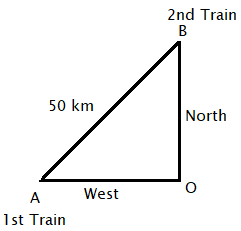By Pythagoras Theorem, we have

AB2 = OA2 + OB2

(50)2 = [2(x + 5)]2 + (2x)2

2500 = 4(x2 + 10x + 25) + 4x2

2500 = 8x2 + 40x + 100

x2 + 5x – 300 = 0

x2 + 20x – 15x – 300 = 0

(x + 20) (x – 15) = 0

So, x = -20 or x = 15

As x cannot be negative, we have x = 15

Thus, the speed of the second train is 15 km/hr and the speed of the first train is 20 km/hr.

8. The sum S of first n even natural numbers is given by the relation S = n(n + 1). Find n, if the sum is 420.

Solution:

Given relation, S = n(n + 1)

And, S = 420

So, n(n + 1) = 420

n2 + n – 420 = 0

n2 + 21n – 20n – 420 = 0

n(n + 21) – 20(n + 21) = 0

(n + 21) (n – 20) = 0

n = -21, 20

As, n cannot be negative.

Therefore, n = 20.

9. The sum of the ages of a father and his son is 45 years. Five years ago, the product of their ages (in years) was 124. Determine their present ages.

Solution:

Let’s assume the present ages of father and his son to be x years and (45 – x) years respectively.

So five years ago,

Father’s age = (x – 5) years

Son’s age = (45 – x – 5) years = (40 – x) years

From the question, the below equation can be formed

(x – 5) (40 – x) = 124

40x – x2 – 200 + 5x = 124

x2 – 45x +324 = 0

x2 – 36x – 9x +324 = 0

x(x – 36) – 9(x – 36) = 0

(x – 36) (x – 9) = 0

x = 36, 9

So, if x = 9,

The father’s age = 9 years and the son’s age = (45 – x) = 36 years

This is not possible.

Hence, x = 36

Therefore,

The father’s age = 36 years

The son’s age = (45 – 36) years = 9 years

The given solutions are as per the 2019-20 Concise Selina textbook. The Selina Solutions for the academic year 2020-21 will be updated soon.